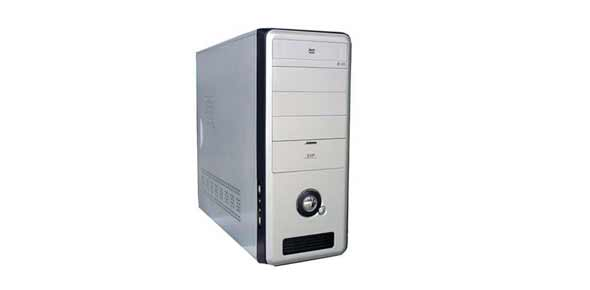# Cop4858 Final Review - 52 Questions

52 Questions | Total Attempts: 103SettingsCreate your own Quiz• 1.
In the decimal or binary notations, the symbol used to represent a digit and the digit’s position in a string determines its ____.
• A.

Sign

• B.

Placement

• C.

Base

• D.

Value

• 2.
____ occurs when the absolute value of a negative exponent is too large to fit in the bits allocated tostore it.
• A.

Rounding

• B.

Rotation

• C.

Overflow

• D.

Underflow

• 3.
A(n) ____ is a set of related data values.
• A.

Pointer

• B.

List

• C.

Array

• D.

Record

• 4.
A(n) ____ is a data structure that uses pointers so that list elements can be scattered among nonsequential storage locations.
• A.

Array

• B.

Queue

• C.

• D.

Stack

• 5.
During the ____ cycle of the CPU, data inputs are prepared for transformation into data outputs.
• A.

Execution

• B.

Store

• C.

Fetch

• D.

Wait

• 6.
A ____ instruction copies data bits to storage locations and can copy data between any combination of registers and primary storage locations.
• A.

JUMP

• B.

• C.

STORE

• D.

MOVE

• 7.
An ____ is a template that specifies the number of operands and the position and length of the op code and operands.
• A.

Instruction format

• B.

Instruction template

• C.

Operation format

• D.

Operand template

• 8.
When manipulating single-precision floating-point numbers, CPU performance is measured in ____.
• A.

Watts

• B.

MHZ

• C.

MFLOPS

• D.

MIPS

• 9.
____ states that the cost of fabrication facilities for the latest chip generation doubles every four years.
• A.

Rick’s Law

• B.

Moore’s Law

• C.

Nelson’s Law

• D.

Lamb’s Law

• 10.
____ is a generic term for describing secondary storage data transfer units.
• A.

Sector

• B.

Wafer

• C.

Cluster

• D.

Block

• 11.
____ stores each bit by using a single transistor and capacitor.
• A.

Dynamic ROM

• B.

Static ROM

• C.

Dynamic RAM

• D.

Static RAM

• 12.
The ratio of cache hits to read accesses is called the cache’s ____.
• A.

Hit ratio

• B.

Efficiency

• C.

Performance

• D.

Hit boundary

• 13.
____ sensors capture input from special-purpose symbols placed on paper or the flat surfaces of 3D objects.
• A.

Dot and image

• B.

Mark and image

• C.

Mark and pattern

• D.

Image capture

• 14.
PDF417 bar codes can hold around ____ of data.
• A.

1 KB

• B.

1 MB

• C.

1 GB

• D.

1 TB

• 15.
A(n) ____ is the maximum number of bits or bytes per second that the channel can carry.
• A.

Minimum data transfer rate

• B.

Overhead data transfer rate

• C.

Net data transfer rate

• D.

Raw data transfer rate

• 16.
As bit transmission speed is increased, the duration of each bit in the signal, known as the ____, decreases.
• A.

Bit time

• B.

• C.

Block time

• D.

Modulation time

• 17.
A(n) ____ extracts the data embedded in the signal it receives and retransmits a new signalcontaining the same data.
• A.

Repeater

• B.

Multiplexer

• C.

Amplifier

• D.

Booster

• 18.
The ____ standard divides frequency bands in the 5.2, 5.7, and 5.8 GHz ranges into 12 channels.
• A.

802.11n

• B.

802.11g

• C.

802.11b

• D.

802.11a

• 19.
The term ____ refers to the spatial organization of network devices, physical routing of network cabling, and flow of messages from one network node to another.
• A.

Network infrastructure

• B.

Network topology

• C.

Network path

• D.

Network interface

• 20.
The ____ layer includes communication protocols used by programs that make and respond to high-level requests for network services.
• A.

Presentation

• B.

• C.

Network

• D.

Application

• 21.
The ____ layer forwards messages to their correct destinations.
• A.

Session

• B.

• C.

Network

• D.

• 22.
The ____ layer is the interface between network software and hardware.
• A.

Session

• B.

• C.

Application

• D.

Network

• 23.
IP accepts messages called ____ from Transport-layer protocols and forwards them to theirdestination.
• A.

Datagrams

• B.

Frames

• C.

Blocks

• D.

Packets

• 24.
The written format of 128-bit IP addresses is called ____ notation.
• A.

Dotted decimal

• B.

• C.

Colon decimal

• D.

• 25.
At the sending node, the ____ layer’s role is to translate IP datagrams into a format that can be transported and forwarded over a specific physical network.
• A.

Application

• B.

Network Interface

• C.

Session

• D.

Transport

Related TopicsBack to top
×

Wait!
Here's an interesting quiz for you.